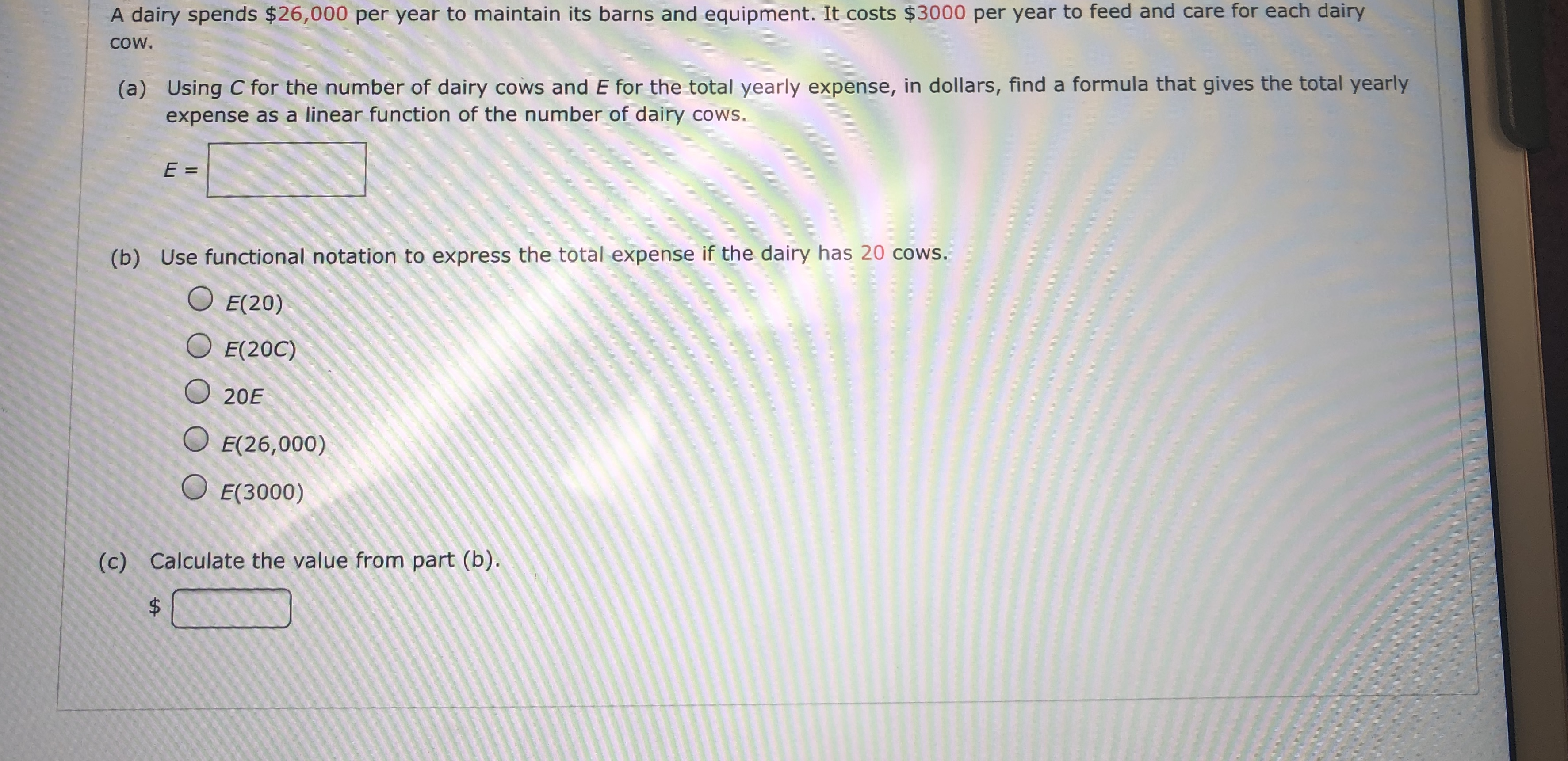A dairy spends \$26,000 per year to maintain its barns and equipment. It costs \$3000 per year to feed and care for each dairyCOW.(a) Using C for the number of dairy cows and E for the total yearly expense, in dollars, find a formula that gives the total yearlyexpense as a linear function of the number of dairy cows.E =(b) Use functional notation to express the total expense if the dairy has 20 cows.OE(20)E(20C)20EE(26,000)E(3000)(c) Calculate the value from part (b).\$tA

Question

What are the blanks?help_outlineImage TranscriptioncloseA dairy spends \$26,000 per year to maintain its barns and equipment. It costs \$3000 per year to feed and care for each dairy COW. (a) Using C for the number of dairy cows and E for the total yearly expense, in dollars, find a formula that gives the total yearly expense as a linear function of the number of dairy cows. E = (b) Use functional notation to express the total expense if the dairy has 20 cows. OE(20) E(20C) 20E E(26,000) E(3000) (c) Calculate the value from part (b). \$ tA fullscreen
Step 1

A diary spends \$26000 per year to maintain its barn and equipment. It costs \$3000 per year to feed and care for each dairy cow.

Let C be the number of dairy cow and E be the total year expensive.

According to the given conditions,

Step 2

The total expensive if the...

Want to see the full answer?

See Solution

Want to see this answer and more?

Our solutions are written by experts, many with advanced degrees, and available 24/7

See Solution
Tagged in

Other